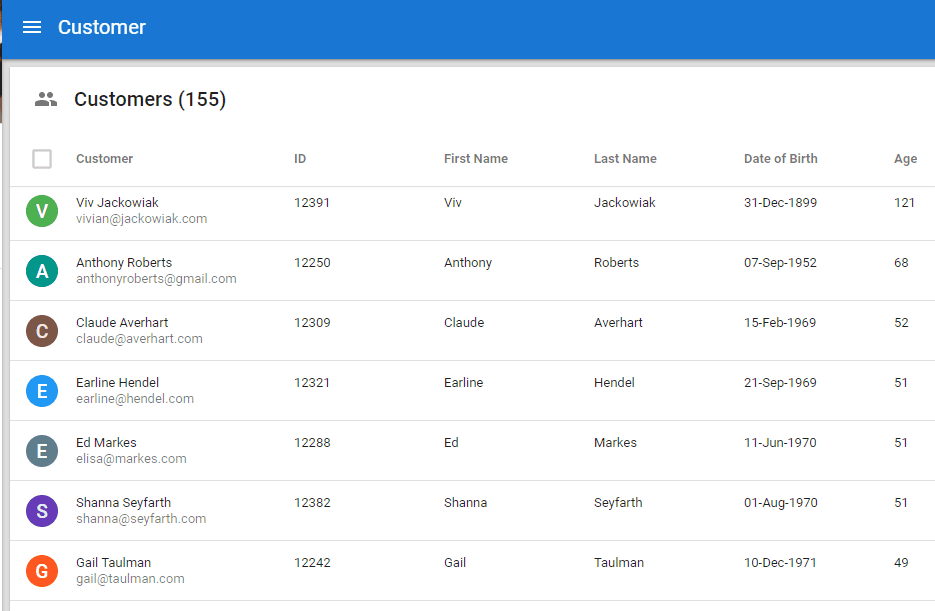Start a new topic

## How do I calculate the Age of a person with a given birthdate and return it as an integer?

How do I calculate the Age of a person with a given birthdate and return it as an integer, I am using Semarchy 5.2 and Postgres as the backend (I want to ensure that people records with date of birth have someone in an allowed age range). I tried something like this but it does not work as an expression in the Validations feature: DATE_PART('year',AGE(CAST('06/06/1966' as TIMESTAMP)))

# Hi BrentYou can use the following SemQL expression:`date_part('year', age(CURRENT_DATE(), DateOfBirth ) )`Put this into a Collection table column labeled 'Age' and you get this:Age as Short Integer Default Value Data Type

The above can be also an Enricher Expression but remember if you need to put it in a validation then it needs to resolve to either a True/False e.g.

` date_part('year', age(CURRENT_DATE(), DateOfBirth ) ) > 65`

1 Comment

# Hi BrentYou can use the following SemQL expression:`date_part('year', age(CURRENT_DATE(), DateOfBirth ) )`Put this into a Collection table column labeled 'Age' and you get this:Age as Short Integer Default Value Data Type

The above can be also an Enricher Expression but remember if you need to put it in a validation then it needs to resolve to either a True/False e.g.

` date_part('year', age(CURRENT_DATE(), DateOfBirth ) ) > 65`